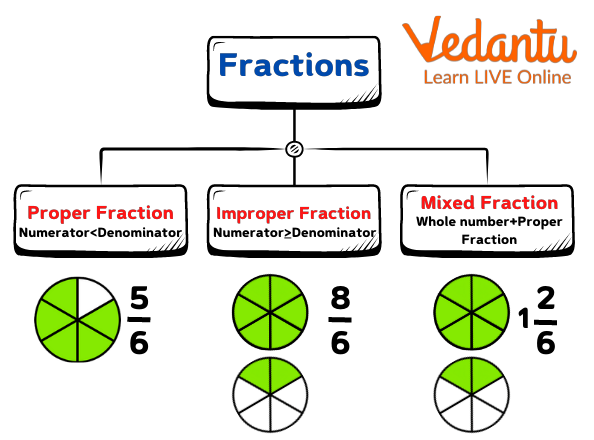Courses
Courses for Kids
Free study material
Free LIVE classes
More

# How Many Types of FractionLIVE
Join Vedantu’s FREE Mastercalss

## An Introduction to Types of Fractions

A fraction is defined as a part or portion of any quantity out of a whole, where the whole can be any number, a specific value, or a thing.

Types of fractions are categorised mostly on the basis of their numerator and denominator. A fraction is composed of two parts; these are the numerator and the denominator. The number placed on the top of the fractional bar is known as the numerator and the number placed at the bottom is known as the denominator. The numerator shows the number of parts that are being considered while the denominator indicates the total number of parts in the whole.

## Types of Fractions with Examples

There are many types of fractions but the three main types of fractions which are differentiated on the basis of the numerator and the denominator are given below.

• Proper fractions

• Improper fractions

• Mixed fractionsLet’s understand each type of fraction in detail.

1. Proper Fractions

A proper fraction is defined as a fraction whose numerator is less than its denominator. The examples of the proper fractions are $\frac{4}{7}$ and $\frac{8}{15}$ because 4 < 7 and 8 < 15. Example: Rohan took a stick and he broke it into 3 equal parts. He took 1 part and gave 2 parts to his friend. We can represent Rohan's portion as 1/3 and his friend’s portion as 2/3. Both of these fractions are proper fractions here.

2. Improper Fractions

An improper fraction is defined as a fraction whose numerator is greater than or equal to its denominator.

For example, $\frac{7}{2}$ and $\frac{8}{15}$ are improper fractions because 7 > 2 and 19>7.

3. Mixed Fractions

A mixed fraction is a combination of a whole number and a proper fraction. For example, $1\frac{4}{9}$ and $2\frac{4}{17}$ are mixed numbers or mixed fractions. In the first example, 1 is the whole number part and $\frac{4}{9}$ is the proper fraction. In the second example, 2 is the whole number part and $1\frac{4}{17}$ is the proper fraction.

## Group of Fractions

There are some types of fractions that are classified into groups. Groups of fractions are helpful in comparing fractions. These are as follows.

• Like fractions

• Unlike fractions

• Equivalent fractions

1.   Like Fractions

In like fractions, the denominators of two or more fractions are the same. For example $\frac{1}{8}$, $\frac{3}{8}$, $\frac{7}{8}$, $\frac{19}{8}$ are like fractions. Mathematical operations such as addition and subtraction can be easily performed on like fractions.

2. Unlike Fractions

In unlike fractions, the denominators of two or more fractions are different. For example $\frac{2}{7}$, $\frac{1}{3}$, $\frac{5}{7}$, $\frac{1}{6}$ etc. In order to perform mathematical operations in unlike fractions we may convert them into like fractions.

3.Equivalent Fractions

When equivalent fractions are simplified or reduced they give the same value while these fractions have different numerators and different denominators. For example, $\frac{12}{24}$, $\frac{6}{12}$, $\frac{4}{8}$ are all equivalent fractions because they all get reduced to $\frac{1}{2}$.

## Important Points

Some important points related to the different types of fractions are given below.

• A mixed fraction is a fraction which is the combination of a fraction and a whole number. The conversion of a mixed fraction can be converted into an improper fraction and vice versa. For example, $3\frac{1}{4}=\frac{3х4+1}{4}=\frac{13}{4}$

• In an improper fraction, we know that the value of a numerator is greater than or equal to its denominator. So its value will always be greater than unity.

• In a proper fraction, the numerator is less than its denominator so its value is always less than unity.

## Do You Know?

Units fractions are those fractions where the value of the numerator is 1 and the denominator is a positive integer. For example,$\frac{1}{3}$, $\frac{1}{8}$, $\frac{1}{19}$, $\frac{1}{23}$ and so on are known as unit fractions.

## Conclusion

Fraction and its types are important and basic concepts of mathematics. In this article, we have learnt about how many types of fractions are there with examples, such as proper and improper fractions, mixed fractions, equivalent fractions, like and unlike fractions.

Last updated date: 23rd Sep 2023
Total views: 138.9k
Views today: 2.38k

## FAQs on How Many Types of Fraction

1. What are the parts of fractions?

There are two parts of fractions; these are the numerator and the denominator. The numerator is the number that is placed on the top of the fraction. It is here, a fraction indicates the part that is considered out of the whole. For example, in $\frac{10}{3}$, 10 is the numerator. And the second part the denominator indicates the part that is placed on the bottom of the fraction. It represents the total number of parts. In $\frac{10}{3}$ , 3 is the denominator.

2. How many types of fractions?

There are seven different types of fractions that are divided into different categories. Fractions can be classified on the basis of the numerator and the denominator. In this way, they are categorised as proper fractions, improper fractions, mixed fractions. Fractions can be classified in groups they are termed as like fractions, unlike fractions and equivalent fractions. Then, there is also one type of fraction which is called unit fraction which has 1 as the numerator.